# Check if a number is an Integer or Float in PythonLast updated: Feb 20, 2023
8 min## #Check if a number is an int or float in Python

Use the `isinstance()` function to check if a number is an int or float.

The `isinstance` function will return `True` if the passed-in object is an instance of the provided class (`int` or `float`).

main.py
```Copied!```my_num = 1357

if isinstance(my_num, int):
print('number is int')

if isinstance(my_num, float):
print('number is float')

# -----------------------------------

# ✅ checks if a number is either int or float

if isinstance(my_num, (int, float)):
print('Number is either int or float')
``````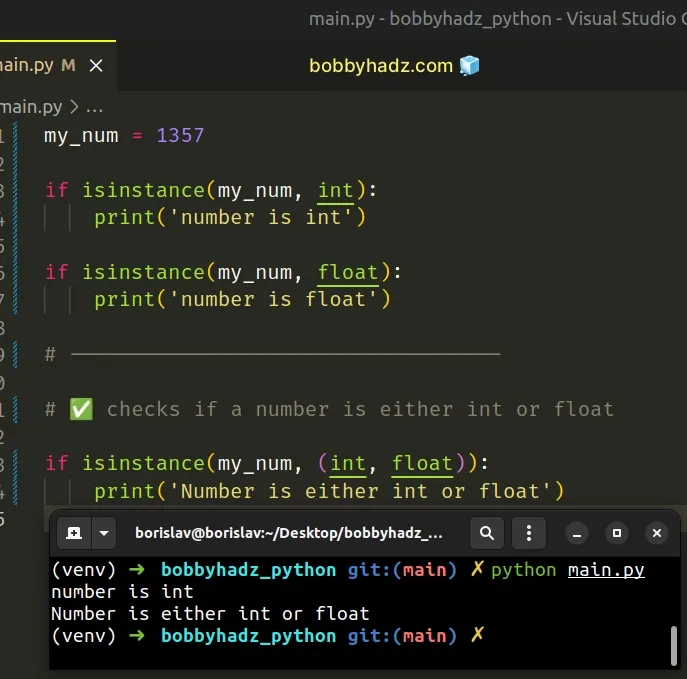We used the `isinstance()` function to check if a number is an `int` or a `float`.

The isinstance function returns `True` if the passed-in object is an instance or a subclass of the passed-in class.

main.py
```Copied!```print(isinstance(357, int))  # 👉️ True
print(isinstance(357, float))  # 👉️ False

print(isinstance(3.14, float))  # 👉️ True
print(isinstance(3.14, int))  # 👉️ False
``````

The `isinstance()` function correctly returns whether the passed-in object is an instance or a subclass of the provided class.

However, there is an edge case - booleans are also an instance of integers.

main.py
```Copied!```print(isinstance(True, int))  # 👉️ True
print(isinstance(False, int))  # 👉️ True
``````

This is because the `bool` class is a subclass of int.

If you need to check if a number is either int or float, pass a tuple containing the `int` and `float` classes in the call to the `isinstance()` function.

main.py
```Copied!```my_num = 1357

if isinstance(my_num, (int, float)):
# 👇️ this runs
print('Number is either int or float')
``````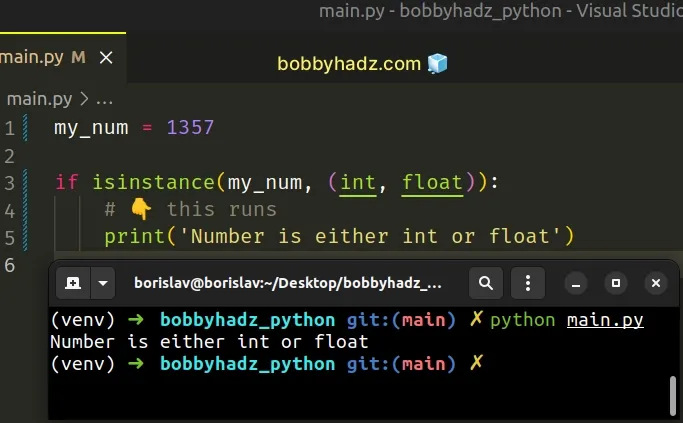The second argument the `isinstance` function takes can be a class or a tuple containing multiple classes.

The call to the function above checks if the passed-in object is either an `int` or a `float`.

Using a `tuple` in the call to the function is equivalent to using two calls with the `OR` operator.

main.py
```Copied!```my_num = 1357

if isinstance(my_num, int) or isinstance(my_num, float):
print('Number is either int or float')
``````

If you just want to print the type of the number, use the `type()` class.

main.py
```Copied!```my_num = 1357
print(type(my_num)) # 👉️ <class 'int'>

my_num_2 = 3.14
print(type(my_num_2)) # 👉️ <class 'float'>
``````

The type class returns the type of an object.

Most commonly the return value is the same as accessing the `__class__` attribute on the object.

## #Check if a string is an Integer or a Float in Python

To check if a string is an integer or a float:

1. Use the `str.isdigit()` method to check if every character in the string is a digit.
2. If the method returns `True`, the string is an integer.
3. If the method returns `False`, the string is a floating-point number.
main.py
```Copied!```my_str = '2468'

if my_str.isdigit():
my_num = int(my_str)
print('String is an integer')
else:
my_num = float(my_str)
print('String is a float')

print(my_num)  # 👉️ 2468
``````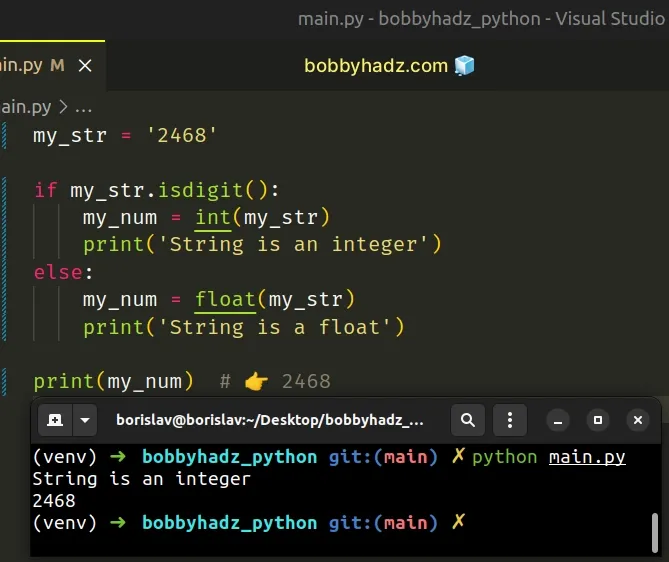If you have to handle negative numbers, scroll down to the `try/except` solution.

We used the `str.isdigit()` method to check if all characters in the string are digits.

The str.isdigit method returns `True` if all characters in the string are digits and there is at least 1 character, otherwise `False` is returned.

The `str.isdigit()` metho would return `False` if the string has a decimal point or starts with a minus `-` (is a negative number).

main.py
```Copied!```print('-123'.isdigit()) # 👉️ False
print('1.23'.isdigit()) # 👉️ False
``````

If you don't have to handle negative numbers, use the `str.isdigit()` method as it is sufficient.

If you have to handle negative numbers, use a try/except block.

## #Check if a string is an Integer or a Float using `try/except`

This is a three-step process:

1. Wrap the call to the `int()` class in a `try` block.
2. If the call to the `int()` class succeeds, the string is an integer.
3. If the `except` block runs, the string is a floating-point number.
main.py
```Copied!```my_str = '-2468'

try:
my_num = int(my_str)

# 👇️ this runs
print('String is an integer')
except ValueError:
my_num = float(my_str)
print('String is a float')

print(my_num) # 👉️ -2468
``````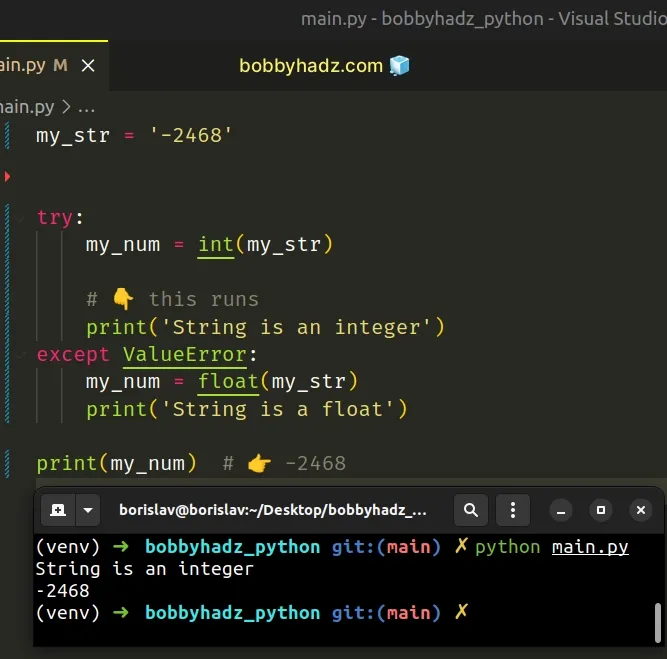We used a `try/except` statement to check if a string is an integer or a float.

If the `try` block runs successfully, the string is an integer.

If calling the `int()` class with the string raises a `ValueError`, the `except` block is run and the string is a floating-point number.

Unlike the `str.isdigit()` method, this approach also handles negative numbers.

Using a `try/except` statement in this manner is commonly known as "asking for forgiveness rather than permission".

We pass the string to the `int()` class not knowing whether the conversion will be successful, and if a `ValueError` error is raised, we handle it in the `except` block.

## #Check if a String can be converted to an Integer in Python

To check if a string can be converted to an integer:

1. Wrap the call to the `int()` class in a `try/except` block.
2. If the conversion succeeds, the `try` block will fully run.
3. If the conversion to integer fails, handle the `ValueError` in the `except` block.
main.py
```Copied!```def can_convert_to_int(string):
try:
int(string)

return True
except ValueError:
return False

print(can_convert_to_int('1357'))  # 👉️ True
print(can_convert_to_int('-1357'))  # 👉️ True
print(can_convert_to_int('1.357'))  # 👉️ False
print(can_convert_to_int('ABC123'))  # 👉️ False
``````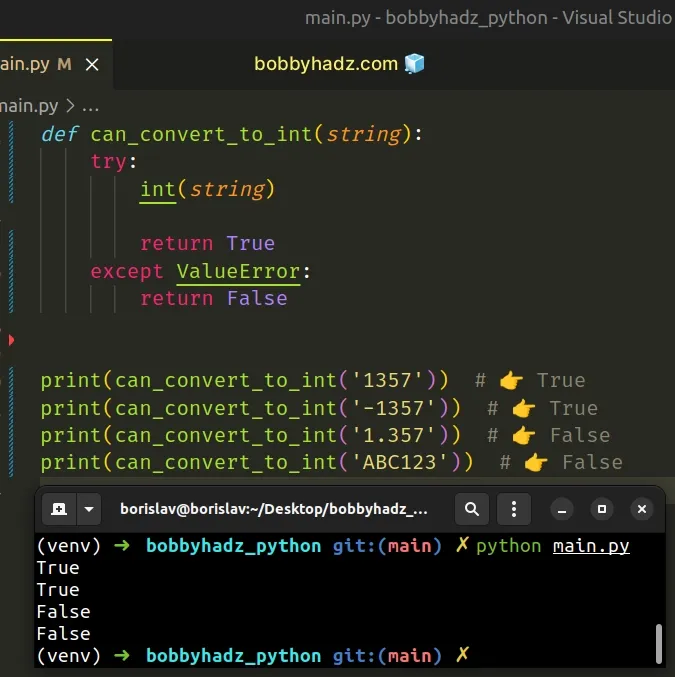We used a `try/except` block to check if a string can be converted to an integer.

If the `try` block succeeds, the function returns `True` and the string can be converted to an integer.

If calling the `int()` class with the string fails, a `ValueError` is raised and the function returns `False`.

Using a `try/except` statement in this manner is commonly known as "asking for forgiveness rather than permission".

We pass the string to the `int()` class not knowing whether the conversion will be successful, and if a `ValueError` error is raised, we handle it in the `except` block.

## #Check if String can be converted to Integer using isdigit()

Alternatively, you can use the `str.isdigit()` method.

If the `str.isdigit` method returns `True`, then all of the characters in the string are digits and it can be converted to an integer.

main.py
```Copied!```my_str = '1357'

if my_str.isdigit():
my_int = int(my_str)
print(my_int)  # 👉️ 1357

print('✅ string can be converted to integer')
else:
print('⛔️ string CANNOT be converted to integer')
``````

We used the `str.isdigit()` method to check if a string can safely be converted to an integer.

The str.isdigit method returns `True` if all characters in the string are digits and there is at least 1 character, otherwise `False` is returned.

The method checks if all characters in the string are digits, so if the string had a minus sign or a decimal, it would return `False`.

main.py
```Copied!```my_str = '-1357'

if my_str.isdigit():
my_int = int(my_str)
print(my_int)

print('✅ string can be converted to integer')
else:
# 👇️ this runs

print('⛔️ string CANNOT be converted to integer')
``````
If your string might be a negative integer, use a `try/except` example instead, as it also covers negative integers.

If you just want to try converting the string to an integer and silence/ignore the `ValueError` if the conversion fails, use a `pass` statement.

main.py
```Copied!```my_str = '2468'

try:
my_int = int(my_str)
print(my_int)  # 👉️ 2468
except ValueError:
pass
``````

The pass statement does nothing and is used when a statement is required syntactically but the program requires no action.

main.py
```Copied!```class Employee:
pass
``````

## #Check if a String can be converted to a Float in Python

To check if a string can be converted to a float:

1. Wrap the call to the `float()` class in a `try/except` block.
2. If the `try` block runs successfully, the string can be converted to a float.
3. If the `except` block runs, the string cannot be converted to a float.
main.py
```Copied!```my_str = '3.456'

try:
result = float(my_str)
print(result)  # 👉️ 3.456
except ValueError:
print('Value cannot be converted to a float')
``````

We used a `try/except` statement to check if a string can be converted to a float.

If the `try` block runs successfully, the string can be converted to a float.

If trying to convert the string to a `float()` raises a `ValueError`, the string cannot be converted to a float.
main.py
```Copied!```my_str = 'abc1.23'

try:
result = float(my_str)
print(result)
except ValueError:
# 👇️ this runs
print('Value cannot be converted to a float')
``````

You can also extract the logic into a reusable function.

main.py
```Copied!```def can_convert_to_float(string):
try:
result = float(string)
print(result)

return True
except ValueError:
return False

print(can_convert_to_float('123'))  # 👉️ True
print(can_convert_to_float('1.23'))  # 👉️ True
print(can_convert_to_float('a.bc'))  # 👉️ False
print(can_convert_to_float('.5'))  # 👉️ True

if can_convert_to_float('3.14'):
# 👇️ this runs
print('String can be converted to a float ✅')
else:
print('String cannot be converted to a float ⛔️')
``````

If the `try` block succeeds, the function returns `True`.

If calling the float() class with the string fails, a `ValueError` is raised and the function returns `False`.

Using a `try/except` statement in this manner is commonly known as "asking for forgiveness rather than permission".

We pass the string to the `float()` class not knowing whether the conversion will be successful, and if a `ValueError` error is raised, we handle it in the `except` block.

If you want to silence/ignore the error when it's raised, use the `pass` statement.

main.py
```Copied!```my_str = 'ab.cd'

try:
result = float(my_str)
print(result)
except ValueError:
pass
``````

The pass statement does nothing and is used when a statement is required syntactically but the program requires no action.

## #Check if a Float is a whole Number in Python

You can use the `float.is_integer()` method to check if a float is a whole number.

main.py
```Copied!```import math

# ✅ check if a float is a whole number using float.is_integer()

result = (3.00).is_integer()
print(result)  # 👉️ True
``````

The first example uses the `float.is_integer()` method to check if a float is a whole number.

The float.is_integer method returns a boolean result:

• `True` if the floating-point number is a finite, whole number
• `False` if it isn't
main.py
```Copied!```print((3.00).is_integer())  # 👉️ True
print((3.14).is_integer())  # 👉️ False
``````

Alternatively, you can use the modulo operator.

## #Check if a float is a whole number using modulo

This is a three-step process:

1. Use the modulo operator to divide the float by `1`.
2. If the remainder from the division is `0`, the number is whole.
3. If the remainder isn't `0`, the number has a decimal point.
main.py
```Copied!```num = 5.00

result = num % 1 == 0
print(result)  # 👉️ True
``````

The modulo (%) operator returns the remainder from the division of the first value by the second.

main.py
```Copied!```print(10 % 1)  # 👉️ 0

print(10.5 % 1)  # 👉️ 0.5
``````

If the remainder we get from dividing the number by `1` is `0`, then we have a whole number.

Conversely, if there is a remainder from dividing by `1`, the number has a decimal point.

Alternatively, you can use the `math.floor()` method.

## #Check if a float is a whole number using math.floor()

This is a three-step process:

1. Pass the number to the `math.floor()` method.
2. The `math.floor()` method will round the number down.
3. Compare the rounded-down number with the number itself.
main.py
```Copied!```import math

num = 7.00

result = math.floor(num) == num
print(result)  # 👉️ True
``````

The math.floor method returns the largest integer less than or equal to the provided number.

main.py
```Copied!```print(math.floor(3.99))  # 👉️ 3
print(math.floor(3.00))  # 👉️ 3
``````

If the rounded-down number is equal to the number itself, then we have a whole number.

If the rounded-down number is different, the number has a decimal point.